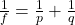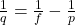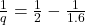## A dentist uses a small concave mirror to locate a cavity in a patient’s tooth. The focal length of the mirror is 2.00 cm. If the mirror is h

Question

A dentist uses a small concave mirror to locate a cavity in a patient’s tooth. The focal length of the mirror is 2.00 cm. If the mirror is held 1.60 cm from the tooth, how many times bigger will the image be? (In other words, what is the magnification?)​

in progress 0
2 weeks 2021-09-03T17:22:19+00:00 1 Answers 0 views 0

m = 4.0

Explanation:

For this exercise in geometric optics we will use the equation of the constructorwhere f is the focal length, p and q are the distance to the object and image, respectively.

In the exercise indicate the focal length f = 2.00 cm, the distance to the object p = 1.60 cm, let’s find the distance to the imagelet’s calculate1 / q = ½ – 1 / 1.6= -0.125

q = -8 cm

the magnification is

m = – q / p

m = 8/2

m = 4.0